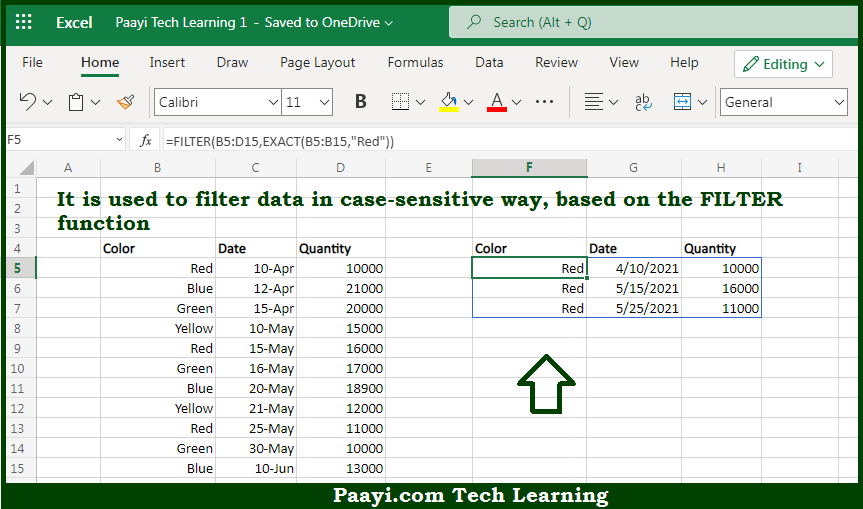# Learn How to Filter With Exact Match in Microsoft Excel

Written by | 0 Comments | 406 Views

In this article, you will learn how to evaluate things in Dynamic Arrays with formulas in Microsoft Excel using a single/combination(s) of functions. You will also know How to Filter With Exact Match and see the generic formula.

Learn How to Filter With Exact Match in Microsoft Excel

The main purpose of this formula is to filter data in a case-sensitive way. Here we will learn how to filter exact matches in the given data range in the workbook in Microsoft Excel. That implies, with the help of a formula based on the FILTER, and EXACT function you can able to filter data in a case-sensitive way. So, with the help of this formula, you can able to filter exact matches in the given data range in the workbook in Microsoft Excel.

General Formula to Filter With Exact Match

=FILTER(data,EXACT (range,A1))

The Explanation for the Filter With Exact MatchSo we know that with the help of the given formula above you can able to filter data in a case-sensitive way. Here we will learn how to filter exact matches in the given data range in the workbook in Microsoft Excel. As we know that the formula relies on the FILTER function to retrieve data based on a logical test. The array argument is provided in B5:D15. That contains all of the data without headers. The "include argument" is an expression based on the EXACT function. So, with the help of this formula, you can able to filter data in a case-sensitive way. Here we will learn how to filter exact matches in the given data range in the workbook in Microsoft Excel.